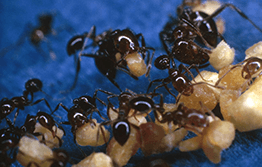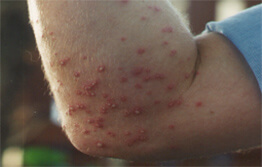```int(43)
bool(false)
int(0)
```
```int(1306)
bool(false)
int(43)
```
```int(2309)
bool(false)
int(43)
```
```int(55)
bool(false)
int(43)
```
```int(1307)
bool(false)
int(43)
```
```int(41)
bool(false)
int(0)
```
```int(60)
bool(false)
int(41)
```
```int(1311)
bool(false)
int(41)
```
```int(1371)
bool(false)
int(41)
```
```int(2576)
bool(false)
int(41)
```
```int(406)
bool(false)
int(41)
```
```int(42)
bool(false)
int(0)
```
```int(1304)
bool(false)
int(42)
```
```int(1303)
bool(false)
int(42)
```
```int(407)
bool(false)
int(42)
```
```int(62)
bool(false)
int(42)
```
```int(51)
bool(false)
int(42)
```
```int(2587)
bool(false)
int(42)
```
```int(3161)
bool(false)
int(42)
```
```int(3163)
bool(false)
int(42)
```
```int(3164)
bool(false)
int(42)
```
```int(3165)
bool(false)
int(42)
```
```int(3166)
bool(false)
int(42)
```
```int(3167)
bool(false)
int(42)
```
```int(3168)
bool(false)
int(42)
```
```int(3169)
bool(false)
int(42)
```
```int(3170)
bool(false)
int(42)
```
```int(3171)
bool(false)
int(42)
```
```int(3172)
bool(false)
int(42)
```
```int(3173)
bool(false)
int(42)
```
```int(3174)
bool(false)
int(42)
```
```int(2951)
bool(false)
int(0)
```
```int(2952)
bool(false)
int(2951)
```
```int(2953)
bool(false)
int(2951)
```
```int(2954)
bool(false)
int(2951)
```
```int(2955)
bool(false)
int(2951)
```Because of their aggression and fiery sting, the establishment of red imported fire ant nests can threaten our outdoor lifestyle, the environment and the production of some agricultural industries. They can also impact on the value of land and businesses.

The ants are 2-6mm long, reddish-brown in colour and very aggressive. They can sting en masse and cause blistering and sometimes an allergic reaction.It’s found in parts of Queensland where they are trying to eradicate it. If you think you have seen red imported fire ants outside of known areas in Queensland and anywhere else in Australia, call 1800 084 881.Red imported fire ants can be spread through the movement of infested materials, such as pot plants, soil, mulch and timber.